Latest Banking jobs   »   Quantitative Aptitude Revision Test For IBPS...

# Quantitative Aptitude Revision Test For IBPS RRB PO, Clerk Prelims 2021- 02nd May

Q1. Abhi invested Rs x at the rate of 15% p.a. on CI for two years and gets total interest of Rs 5805. If Abhi invested Rs (x + 14000) for another 2 yrs at an additional rate of 15%, then what will be the CI on that investment?
(a) Rs 22080
(b) Rs 24000
(c) Rs 25200
(d) Rs 26080
(e) Rs 20080

Q2. Three friends P, Q and R have amount in the ratio of 5 : 6 : 8. If R gives 37½% of his amount to P then the difference of the amount left with R and the average of the amount of P and Q is Rs 560. Find the ratio of their initial amount after Rs 1000 is added to each of them.
(a) 60 : 66 : 83
(b) 67 : 72 : 81
(c) 60 : 67 : 81
(d) 60 : 69 : 81
(e) 65 : 71 : 83

Q3. Trains A and B are travelling at x km/hr and (x + 36) km/hr respectively. Train B crosses train A when running in the same direction in 31½ seconds. Find the sum of speed of both the trains if they pass each other in 9 seconds while running in opposite direction
(a) 126 km/hr
(b) 120 km/hr
(c) 116 km/hr
(d) 136 km/hr
(e) 115 km/hr

Q4. Neeraj invested Rs 10,000 at rate of interest 20% per annum. The interest was compounded yearly for the first two years and in the third year it was compounded half yearly. What will be the total interest earned at the end of the third year?
(a) Rs 7224
(b) Rs 7324
(c) Rs 7424
(d) Rs 7624
(e) Rs 7824

Q5. Find sum of money invested by a man in scheme which offers CI at 5% rate of interest per annum if CI for 2nd year is Rs. 3150
(a) Rs. 55,000
(b) Rs. 30,000
(c) Rs. 40,000
(d) Rs. 60,000
(e) Rs. 65,000

Q6. A natural number when increased by 50% it gives a natural number. However, when the value of the number is reduced by 75%, the number is still natural. If on reducing the number by 66⅔ %, number is still natural than least number that could be –
(a) 6
(b) 18
(c) 12
(d) 24
(e) 30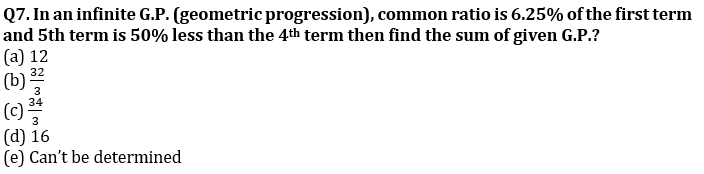Q8. A sum of Rs. 54.60 is comprised of 153 coins that are either 20 paise or 50 paise. Find the number of 20 paise coins
(a) 80
(b) 43
(c) 27
(d) 63
(e) None of these

Q9. Ram invested Rs. 30000 at the rate of X% for two years on compound interest and gets total amount of Rs. 39675. If Ram invested same amount at the rate of (X + 5)% for two years on compound interest, then find how much interest will Ram get?
(a) 12600 Rs.
(b) 14400 Rs.
(c) 13200 Rs.
(d) 12800 Rs.
(e) 16400 Rs.

Total 846 people watching movie in a multiplex ‘P’. Number of adult male who watching movie is 70% more than number of male child, while number of adult female are 60 more than number of male child. Number of female child is 33⅓% less than that of male child.

Q10. Total adult male who watching movie is what percent more than adult female who watching movie in multiplex ‘P’?
(a) 29.5%
(b) 27.5%
(c) 25.5%
(d) 24.5%
(e) 32.5%

Q11. Find average of male child & female child who watching movie in multiplex ‘P’?
(a) 130
(b) 110
(c) 150
(d) 160
(e) 180

Q12. If another multiplex ‘Q’ number of adult female who watching movie is 40% more than male child who watching movie in multiplex ‘P’ and number of adult female who watching movie in multiplex ‘Q’ is 36% of total people watching movie in that multiplex. Find total people watching movie in multiplex ‘P’ is how much more than total people watching movie in multiplex ‘Q’?
(a) 126
(b) 116
(c) 106
(d) 146
(e) 176

Q13. Two trains are travelling with speed 144km/hr and 108 km/hr crosses each other in 6 sec while travelling in opposite direction. The longer train whose length is 60 m more then smaller train and running with speed of 108 km/hr crosses a railway platform in 20 sec. Find the time taken by the smaller train to cross the same platform.
(a) 13.5 sec
(b) 11.5 sec
(c) 12.5 sec
(d) 14.5 sec
(e) 18.5 sec

Q14. Ratio between principle to C.I. for two years is 25 :11. Find the principle if difference between CI & SI for two years is given as Rs 240?
(a) Rs 6000
(b) Rs 5400
(c) Rs 6400
(d) Rs 5000
(e) Rs 4000

Q15. A bird covers a distance of 112 km in ‘y’ hours travelling at the speed of ‘x’ km/hr. Find the speed of bird, given that x and y are co-primes integers, and both are greater than 1.
(a) 16 km/hr
(b) Can’t be determined
(c) 14 km/hr
(d) 28 km/hr
(e) 7 km/hr

Q16. A man borrowed Rs. 10000 from his friend at the rate of 5% p.a. for three years at S.I. Out of total amount he borrowed he invested 80% of amount at the rate of R% p.a. on compound interest for three years and rest he spend. If after three year man still has to pay 852 as interest to his friend, then find ‘R’?
(a) 10%
(b) 5%
(c) 8%
(d) 12%
(e) 15%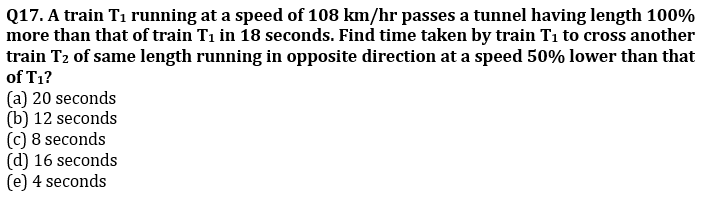Q18. Speed of Satish is 40% of speed of Aman. Aman covers 2340 m in 18 seconds. Find in how much time Satish can cover 468 m.
(a) 8 seconds
(b) 9 seconds
(c) 10 seconds
(d) 11 seconds
(e) 12 seconds

Q19. A sum of money fetches Rs 240 as simple interest at the rate of 5 p.c.p.a. after 6 years. What is the principal?
(a) Rs 200
(b) Rs 400
(c) Rs 800
(d) Rs 1,200
(e) Rs 1,000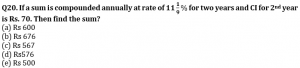Practice More Questions of Quantitative Aptitude for Competitive Exams:

###### Study Plan for IBPS RRB PO/Clerk Prelims 2021

Solutions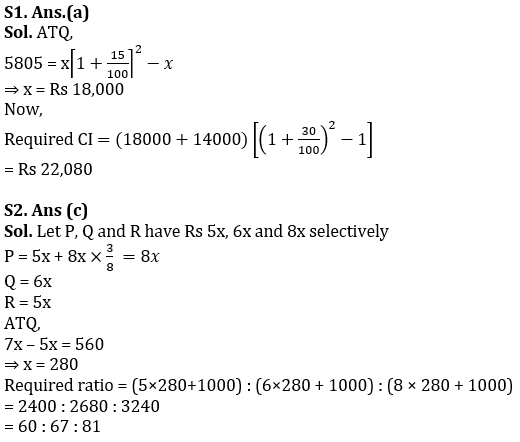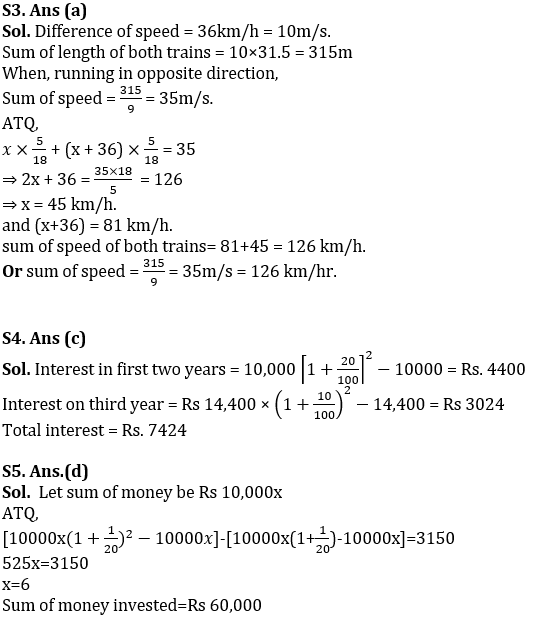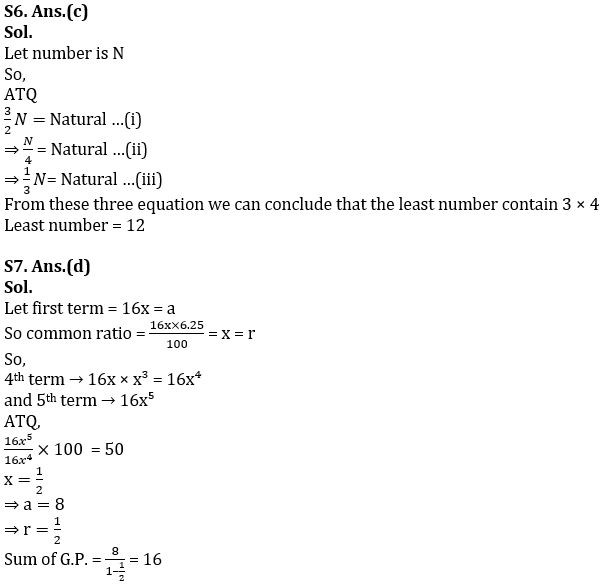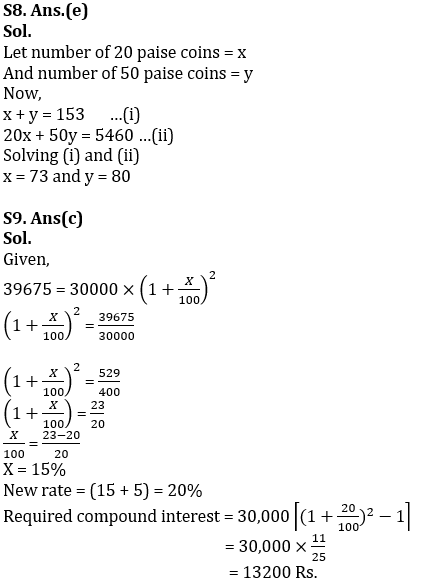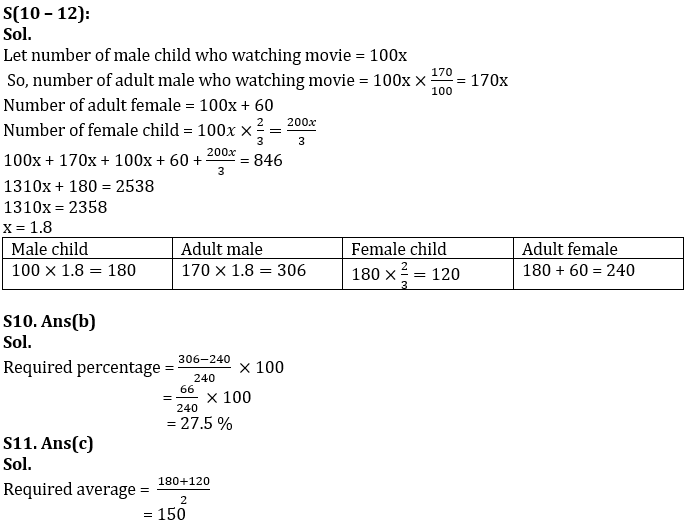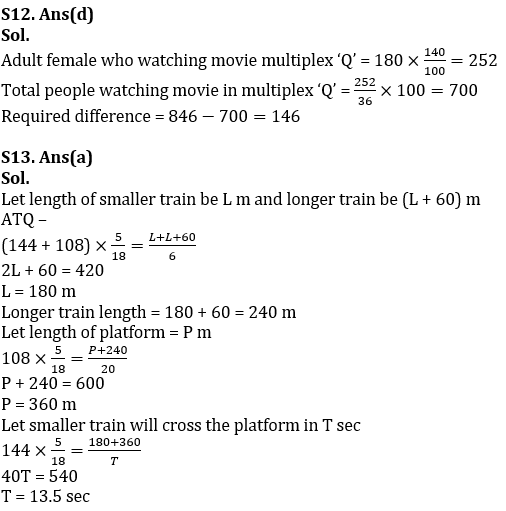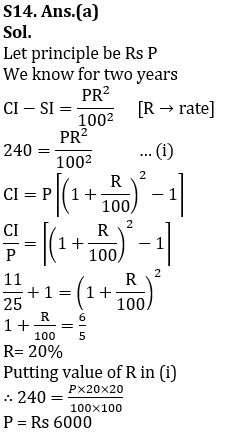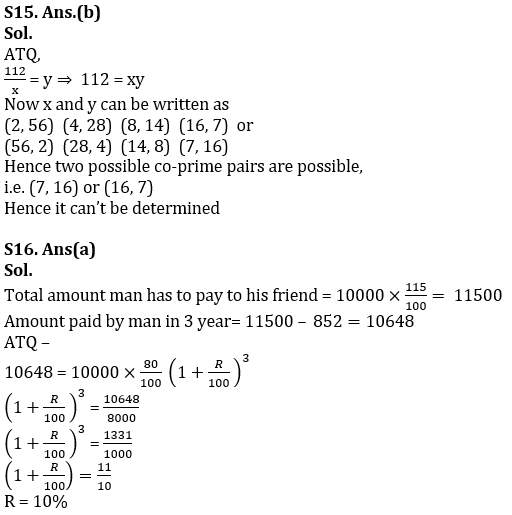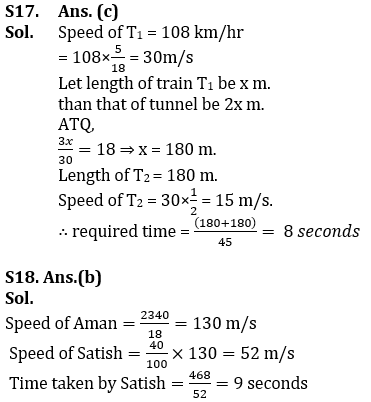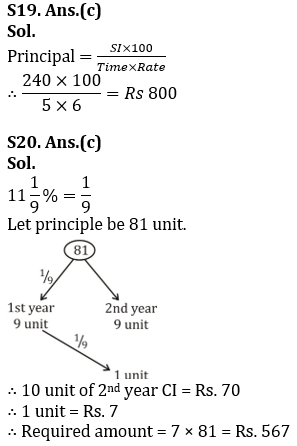#### Congratulations!Incorrect details? Fill the form again here

•English Quizzes For IBPS Clerk/PO Prelim...
•English Quizzes For IBPS Clerk/PO Prelim...
•Quantitative Aptitude Quiz For IBPS Cler...
•Quantitative Aptitude Quiz For IBPS Cler...
•Reasoning Ability Quiz For IBPS Clerk/PO...
•Quantitative Aptitude Quiz For IBPS Cler...